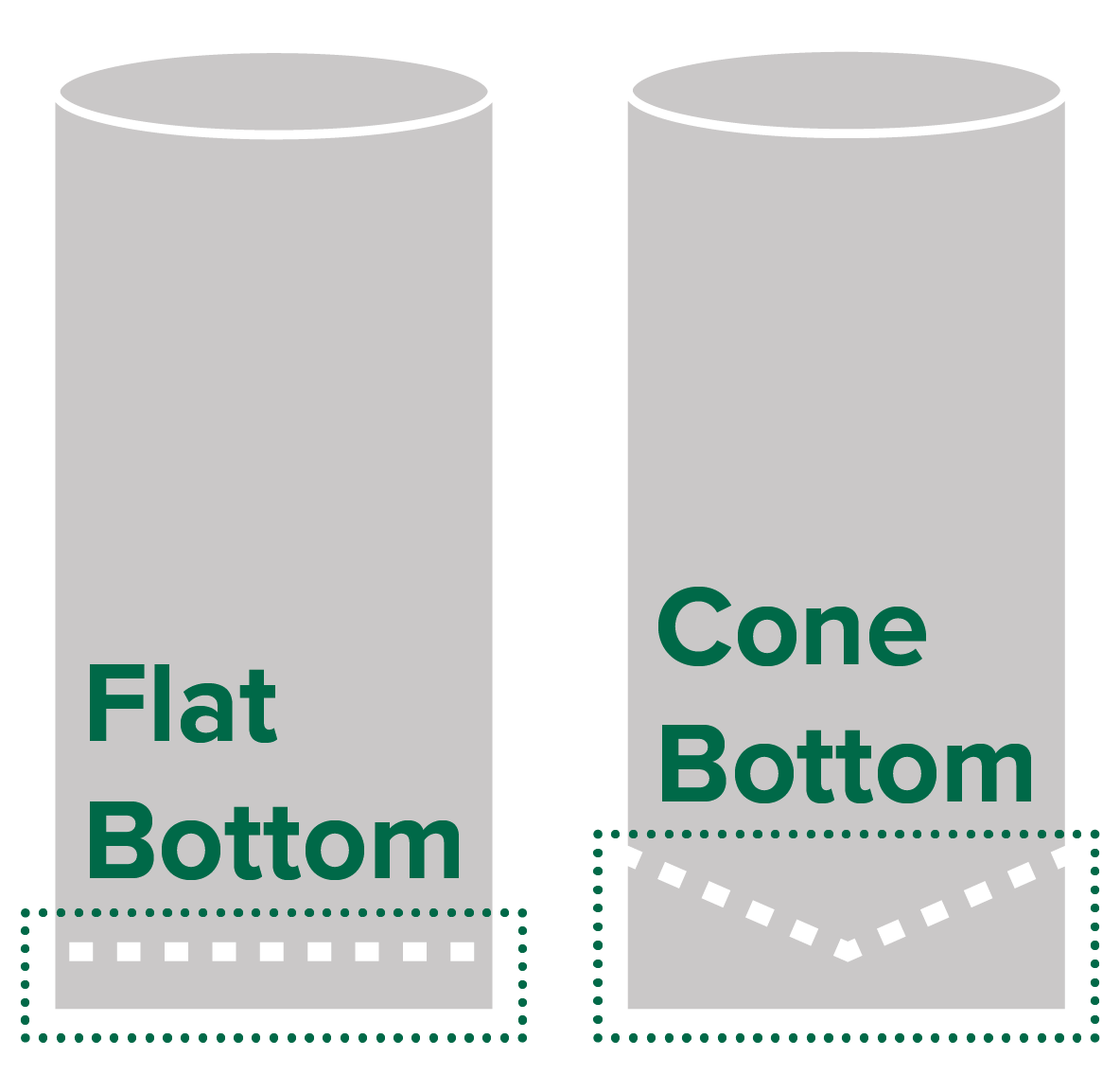# Fiberglass Tanks Calculators

## Custom

### Results

We understand that it might be a challenge to estimate how many gallons are in a container. That’s why we’ve created an easy to use calculator to help you get a better idea on how much liquid is in your tank.

Our calculators can be used to estimate how many gallons are in your Precision Tank storage container and we’ve also created a calculator where you can estimate the amount of liquid for any flat bottom tank.

But first, let’s go over the basic dimensions and numbers needed to calculate the amount of liquid in your tank.

Tank Volume Formula
The total volume of a cylindrical tank is calculated by using the formula Volume = π x Radius2 x Length where Radius = Diameter/2

By entering the Fluid Depth (in inches), represented by the letter "F" in the illustration above, we can calculate an estimated amount of liquid.Precision Tank Types
Precision Tank cylindrical storage tanks can be manufactured with a flat bottom or a cone bottom in a variety of different sizes. To estimate how much liquid is in your Precision Tank tank, simply select your tank’s diameter size from the dropdown list above and then tell us if your tank is a flat bottom or a cone bottom.